2397字，阅读需时8分钟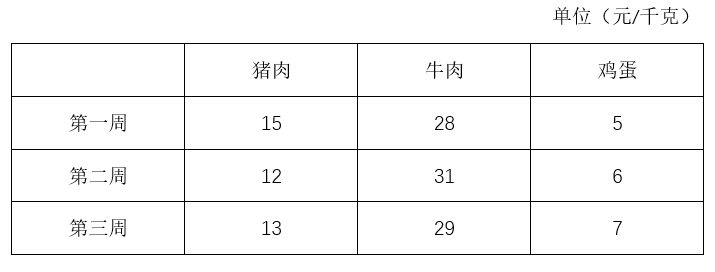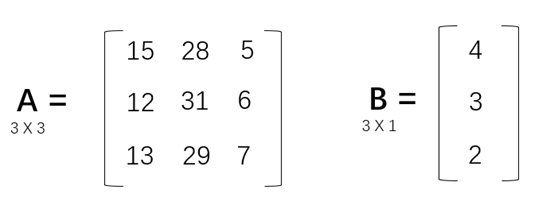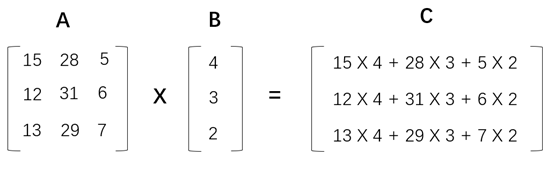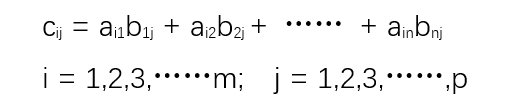A矩阵和B矩阵可以相乘的条件是：A矩阵的列数等于B矩阵的行数。矩阵的乘法不满足交互律，即A X B != B X A。

15x + 28y + 5z = 154

12x + 31y + 6z = 153

13x + 29y + 7z = 153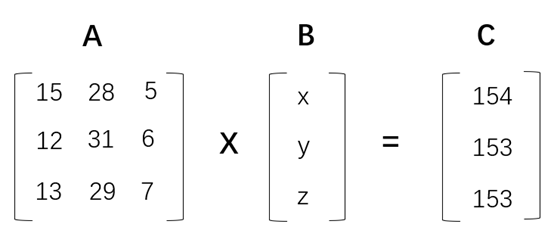15x + 28y + 5z = 154

12x + 31y + 6z = 153

13x + 29y + 7z = 153```#定义矩阵A和B
A = [[15,28,5],[12,31,6],[13,29,7]]
B = [,,]

#定义矩阵乘法运算函数
def matrixMul(A, B):
#创建矩阵C，矩阵C的默认元素都为0
#矩阵C的行数为A矩阵的行数，列数为B矩阵的列数
C = [ * len(B) for i in range(len(A))]
#按行遍历A矩阵
for i in range(len(A)):
#按列遍历B矩阵
for j in range(len(B)):
#C矩阵的第i行第j列所对应的数值
#等于A矩阵的第i行分别乘以B矩阵的第j列之和
for k in range(len(B)):
C[i][j] += A[i][k] * B[k][j]
return C

if __name__ == '__main__':

C = matrixMul(A,B)
print(C)```

```import numpy as np

#定义矩阵A和B
A = np.array([[15,28,5],[12,31,6],[13,29,7]])
B = np.array([,,])

#定义矩阵乘法运算函数
def matrixMul(A, B):
#NumPy的matmul完成矩阵乘法运算
C = np.matmul(A,B)
return C

if __name__ == '__main__':
C = matrixMul(A,B)
print(C)```